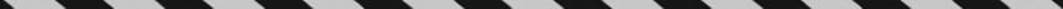﻿ ﻿A FEW MISCELLANEOUS PROBLEMS - Word Problems - ALGEBRA - SAT SUBJECT TEST MATH LEVEL 1

## ALGEBRA## CHAPTER 7Word Problems### A FEW MISCELLANEOUS PROBLEMS

EXAMPLE 6: Adam has five times as many baseball cards as Noah. If Adam gives Noah 200 cards, Noah will have five times as many cards as Adam. How many cards does each of them have?

If Noah now has x cards, Adam has 5x. After Adam gives Noah 200 cards, he will have 5x – 200 and Noah will have x + 200. So:Noah now has 50 cards and Adam has 250 cards.

EXAMPLE 7: An amusement park has two payment options. By using Plan A, you pay a \$10 admission fee plus \$3 for every ride you go on. By using Plan B, you pay a \$20 admission fee and \$1 per ride. What is the least number of rides you must go on for Plan B to be less expensive?

Let x = number of rides you go on. Then the cost using Plan A is 10 + 3x, and the cost using Plan B is 20 + x. SoSo you have to go on at least 6 rides for Plan B to be less expensive.

EXAMPLE 8: In 2000, the ratio of boys to girls performing community service at Central High School was 3 to 5. In 2001, the number of boys increased by 6 and the number of girls increased by 2. If the ratio of boys to girls performing community service in 2001 was 2 to 3, how many students performed community service in 2000?

Let 3x and 5x represent the number of boys and girls doing community service in 2000, respectively. Then in 2001, the numbers were 3x + 6 and 5x + 2. SoSo in 2000, there were 3(14) = 42 boys and 5(14) = 70 girls. The total number of students was 42 + 70 = 112.

EXAMPLE 9: A concession stand sells soda in two sizes: small and large. A small soda costs \$1.50, and a large soda costs \$2.50. One day 200 sodas were sold for a total of \$360. How many small sodas were sold?

If x = the number of small sodas, and y = the number of large sodas, then

x + y = 200 and 1.50x + 2.50y = 360, so replace y by 200 – x in the second equation:﻿